## July 31, 2017

### A Compositional Framework for Reaction Networks

#### Posted by John BaezFor a long time Blake Pollard and I have been working on ‘open’ chemical reaction networks: that is, networks of chemical reactions where some chemicals can flow in from an outside source, or flow out. The picture to keep in mind is something like this: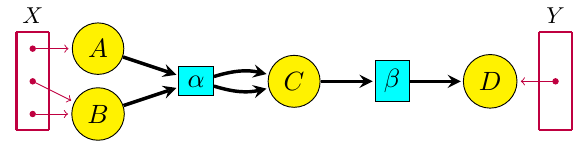where the yellow circles are different kinds of chemicals and the aqua boxes are different reactions. The purple dots in the sets X and Y are ‘inputs’ and ‘outputs’, where certain kinds of chemicals can flow in or out.

Our paper on this stuff just got accepted, and it should appear soon:

But thanks to the arXiv, you don’t have to wait: beat the rush, click and download now!

Or at least read the rest of this blog post….

Blake and I gave talks about this stuff in Luxembourg this June, at a nice conference called Dynamics, thermodynamics and information processing in chemical networks. So, if you’re the sort who prefers talk slides to big scary papers, you can look at those:

But I want to say here what we do in our paper, because it’s pretty cool, and it took a few years to figure it out. To get things to work, we needed my student Brendan Fong to invent the right category-theoretic formalism: ‘decorated cospans’. But we also had to figure out the right way to think about open dynamical systems!

In the end, we figured out how to first ‘gray-box’ an open reaction network, converting it into an open dynamical system, and then ‘black-box’ it, obtaining the relation between input and output flows and concentrations that holds in steady state. The first step extracts the dynamical behavior of an open reaction network; the second extracts its static behavior. And both these steps are functors! So, we’re applying Lawvere’s ideas on functorial semantics to chemistry.

Now Blake has passed his thesis defense based on this work, and he just needs to polish up his thesis a little before submitting it. This summer he’s doing an internship at the Princeton branch of the engineering firm Siemens. He’s working with Arquimedes Canedo on ‘knowledge representation’.

But I’m still eager to dig deeper into open reaction networks. They’re a small but nontrivial step toward my dream of a mathematics of living systems. My working hypothesis is that living systems seem ‘messy’ to physicists because they operate at a higher level of abstraction. That’s what I’m trying to explore.

Here’s the idea of our paper.

### The idea

Reaction networks are a very general framework for describing processes where entities interact and transform int other entities. While they first showed up in chemistry, and are often called ‘chemical reaction networks’, they have lots of other applications. For example, a basic model of infectious disease, the ‘SIRS model’, is described by this reaction network:

$S + I \stackrel{\iota}{\longrightarrow} 2 I \qquad I \stackrel{\rho}{\longrightarrow} R \stackrel{\lambda}{\longrightarrow} S$

We see here three types of entity, called species:

• $S$: susceptible,
• $I$: infected,
• $R$: resistant.

We also have three `reactions’:

• $\iota : S + I \to 2 I$: infection, in which a susceptible individual meets an infected one and becomes infected;
• $\rho : I \to R$: recovery, in which an infected individual gains resistance to the disease;
• $\lambda : R \to S$: loss of resistance, in which a resistant individual becomes susceptible.

In general, a reaction network involves a finite set of species, but reactions go between complexes, which are finite linear combinations of these species with natural number coefficients. The reaction network is a directed graph whose vertices are certain complexes and whose edges are called reactions.

If we attach a positive real number called a rate constant to each reaction, a reaction network determines a system of differential equations saying how the concentrations of the species change over time. This system of equations is usually called the rate equation. In the example I just gave, the rate equation is

$\begin{array}{ccl} \displaystyle{\frac{d S}{d t}} &=& r_\lambda R - r_\iota S I \\ \\ \displaystyle{\frac{d I}{d t}} &=& r_\iota S I - r_\rho I \\ \\ \displaystyle{\frac{d R}{d t}} &=& r_\rho I - r_\lambda R \end{array}$

Here $r_\iota, r_\rho$ and $r_\lambda$ are the rate constants for the three reactions, and $S, I, R$ now stand for the concentrations of the three species, which are treated in a continuum approximation as smooth functions of time:

$S, I, R: \mathbb{R} \to [0,\infty)$

The rate equation can be derived from the law of mass action, which says that any reaction occurs at a rate equal to its rate constant times the product of the concentrations of the species entering it as inputs.

But a reaction network is more than just a stepping-stone to its rate equation! Interesting qualitative properties of the rate equation, like the existence and uniqueness of steady state solutions, can often be determined just by looking at the reaction network, regardless of the rate constants. Results in this direction began with Feinberg and Horn’s work in the 1960’s, leading to the Deficiency Zero and Deficiency One Theorems, and more recently to Craciun’s proof of the Global Attractor Conjecture.

In our paper, Blake and I present a ‘compositional framework’ for reaction networks. In other words, we describe rules for building up reaction networks from smaller pieces, in such a way that its rate equation can be figured out knowing those those of the pieces. But this framework requires that we view reaction networks in a somewhat different way, as ‘Petri nets’.

Petri nets were invented by Carl Petri in 1939, when he was just a teenager, for the purposes of chemistry. Much later, they became popular in theoretical computer science, biology and other fields. A Petri net is a bipartite directed graph: vertices of one kind represent species, vertices of the other kind represent reactions. The edges into a reaction specify which species are inputs to that reaction, while the edges out specify its outputs.

You can easily turn a reaction network into a Petri net and vice versa. For example, the reaction network above translates into this Petri net: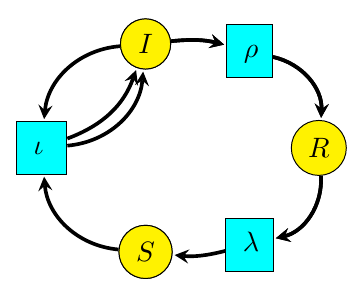Beware: there are a lot of different names for the same thing, since the terminology comes from several communities. In the Petri net literature, species are called places and reactions are called transitions. In fact, Petri nets are sometimes called ‘place-transition nets’ or ‘P/T nets’. On the other hand, chemists call them ‘species-reaction graphs’ or ‘SR-graphs’. And when each reaction of a Petri net has a rate constant attached to it, it is often called a ‘stochastic Petri net’.

While some qualitative properties of a rate equation can be read off from a reaction network, others are more easily read from the corresponding Petri net. For example, properties of a Petri net can be used to determine whether its rate equation can have multiple steady states.

Petri nets are also better suited to a compositional framework. The key new concept is an ‘open’ Petri net. Here’s an example: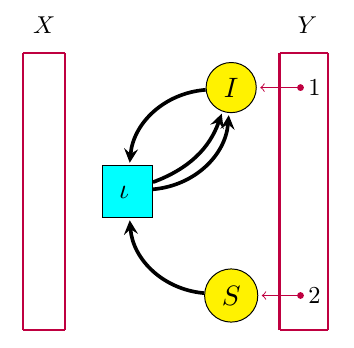The box at left is a set X of ‘inputs’ (which happens to be empty), while the box at right is a set Y of ‘outputs’. Both inputs and outputs are points at which entities of various species can flow in or out of the Petri net. We say the open Petri net goes from X to Y. In our paper, we show how to treat it as a morphism $f : X \to Y$ in a category we call ${RxNet}$.

Given an open Petri net with rate constants assigned to each reaction, our paper explains how to get its ‘open rate equation’. It’s just the usual rate equation with extra terms describing inflows and outflows. The above example has this open rate equation:

$\begin{array}{ccr} \displaystyle{\frac{d S}{d t}} &=& - r_\iota S I - o_1 \\ \\ \displaystyle{\frac{d I}{d t}} &=& r_\iota S I - o_2 \end{array}$

Here $o_1, o_2 : \mathbb{R} \to \mathbb{R}$ are arbitrary smooth functions describing outflows as a function of time.

Given another open Petri net $g: Y \to Z,$ for example this: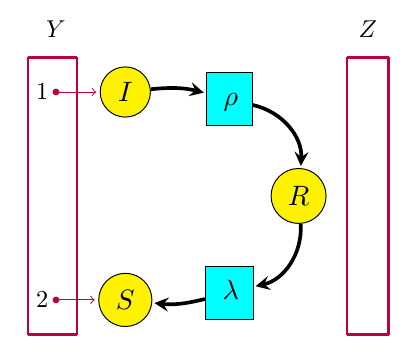it will have its own open rate equation, in this case

$\begin{array}{ccc} \displaystyle{\frac{d S}{d t}} &=& r_\lambda R + i_2 \\ \\ \displaystyle{\frac{d I}{d t}} &=& - r_\rho I + i_1 \\ \\ \displaystyle{\frac{d R}{d t}} &=& r_\rho I - r_\lambda R \end{array}$

Here $i_1, i_2: \mathbb{R} \to \mathbb{R}$ are arbitrary smooth functions describing inflows as a function of time. Now for the first bit of category theory: we can compose $f$ and $g$ by gluing the outputs of $f$ to the inputs of $g.$ This gives a new open Petri net $g f: X \to Z,$ as follows: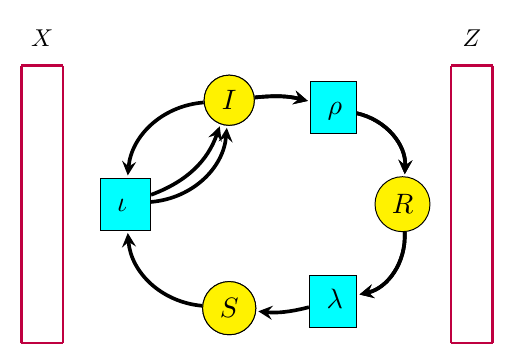But this open Petri net $g f$ has an empty set of inputs, and an empty set of outputs! So it amounts to an ordinary Petri net, and its open rate equation is a rate equation of the usual kind. Indeed, this is the Petri net we have already seen.

As it turns out, there’s a systematic procedure for combining the open rate equations for two open Petri nets to obtain that of their composite. In the example we’re looking at, we just identify the outflows of $f$ with the inflows of $g$ (setting $i_1 = o_1$ and $i_2 = o_2$) and then add the right hand sides of their open rate equations.

The first goal of our paper is to precisely describe this procedure, and to prove that it defines a functor

$\diamond: {RxNet} \to {Dynam}$

from ${RxNet}$ to a category ${Dynam}$ where the morphisms are ‘open dynamical systems’. By a dynamical system, we essentially mean a vector field on $\mathbb{R}^n,$ which can be used to define a system of first-order ordinary differential equations in $n$ variables. An example is the rate equation of a Petri net. An open dynamical system allows for the possibility of extra terms that are arbitrary functions of time, such as the inflows and outflows in an open rate equation.

In fact, we prove that ${RxNet}$ and ${Dynam}$ are symmetric monoidal categories and that $d$ is a symmetric monoidal functor. To do this, we use Brendan Fong’s theory of ‘decorated cospans’.

Decorated cospans are a powerful general tool for describing open systems. A cospan in any category is just a diagram like this: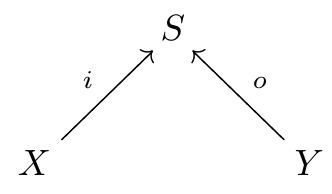We are mostly interested in cospans in ${FinSet},$ the category of finite sets and functions between these. The set $S$, the so-called apex of the cospan, is the set of states of an open system. The sets $X$ and $Y$ are the inputs and outputs of this system. The legs of the cospan, meaning the morphisms $i: X \to S$ and $o: Y \to S,$ describe how these inputs and outputs are included in the system. In our application, $S$ is the set of species of a Petri net.

For example, we may take this reaction network:

$A+B \stackrel{\alpha}{\longrightarrow} 2C \quad \quad C \stackrel{\beta}{\longrightarrow} D$

treat it as a Petri net with $S = \{A,B,C,D\}$: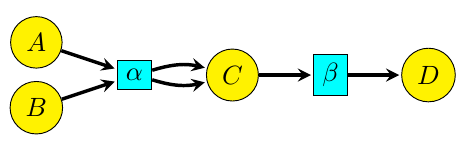and then turn that into an open Petri net by choosing any finite sets $X,Y$ and maps $i: X \to S$, $o: Y \to S$, for example like this:(Notice that the maps including the inputs and outputs into the states of the system need not be one-to-one. This is technically useful, but it introduces some subtleties that I don’t feel like explaining right now.)

An open Petri net can thus be seen as a cospan of finite sets whose apex $S$ is ‘decorated’ with some extra information, namely a Petri net with $S$ as its set of species. Fong’s theory of decorated cospans lets us define a category with open Petri nets as morphisms, with composition given by gluing the outputs of one open Petri net to the inputs of another.

We call the functor

$\diamond: {RxNet} \to {Dynam}$

gray-boxing because it hides some but not all the internal details of an open Petri net. (In the paper we draw it as a gray box, but that’s too hard here!)

We can go further and black-box an open dynamical system. This amounts to recording only the relation between input and output variables that must hold in steady state. We prove that black-boxing gives a functor

$\blacksquare: {Dynam} \to {SemiAlgRel}$

Here ${SemiAlgRel}$ is a category where the morphisms are semi-algebraic relations between real vector spaces, meaning relations defined by polynomials and inequalities. This relies on the fact that our dynamical systems involve algebraic vector fields, meaning those whose components are polynomials; more general dynamical systems would give more general relations.

That semi-algebraic relations are closed under composition is a nontrivial fact, a spinoff of the Tarski–Seidenberg theorem. This says that a subset of $\mathbb{R}^{n+1}$ defined by polynomial equations and inequalities can be projected down onto $\mathbb{R}^n$, and the resulting set is still definable in terms of polynomial identities and inequalities. This wouldn’t be true if we didn’t allow inequalities. It’s neat to see this theorem, important in mathematical logic, showing up in chemistry!

### Structure of the paper

Okay, now you’re ready to read our paper! Here’s how it goes:

In Section 2 we review and compare reaction networks and Petri nets. In Section 3 we construct a symmetric monoidal category ${RNet}$ where an object is a finite set and a morphism is an open reaction network (or more precisely, an isomorphism class of open reaction networks). In Section 4 we enhance this construction to define a symmetric monoidal category ${RxNet}$ where the transitions of the open reaction networks are equipped with rate constants. In Section 5 we explain the open dynamical system associated to an open reaction network, and in Section 6 we construct a symmetric monoidal category ${Dynam}$ of open dynamical systems. In Section 7 we construct the gray-boxing functor

$\diamond: {RxNet} \to {Dynam}$

In Section 8 we construct the black-boxing functor

$\blacksquare: {Dynam} \to {SemiAlgRel}$

We show both of these are symmetric monoidal functors.

Finally, in Section 9 we fit our results into a larger ‘network of network theories’. This is where various results in various papers I’ve been writing in the last few years start assembling to form a big picture! But this picture needs to grow….

Posted at July 31, 2017 3:31 AM UTC

TrackBack URL for this Entry:   https://golem.ph.utexas.edu/cgi-bin/MT-3.0/dxy-tb.fcgi/2973

### Re: A Compositional Framework for Reaction Networks

The purple dots in the sets $X$ and $Y$ are ‘inputs’ and ‘outputs’, where certain kinds of chemicals can flow in or out.

the box at right is a set $Y$ of ‘outputs’

Why do the rightmost arrows point from $Y$ into the main diagram, if they’re outputs?

Posted by: Tom Leinster on July 31, 2017 4:50 PM | Permalink | Reply to this

### Re: A Compositional Framework for Reaction Networks

I figured someone would ask that. The purple arrows just indicate maps from the sets $X$ and $Y$ to the set $S$: we have a cospan

$X \stackrel{i}{\longrightarrow} S \stackrel{o}{\longleftarrow} Y$

The situation is entirely symmetrical under switching the two legs of this cospan, so instead of the words ‘inputs’ and ‘outputs’ it might be better to say ‘leftputs’ and ‘rightputs’.

It’s like a length of pipe: we can formally call one end the ‘input’ end and the other end the ‘output’ end, but the pipe doesn’t know that: water can flow through the pipe in either direction. The main reason for these designations is to remember that when we screw together two pipes, we attach the output of the first to the input of the second.

Similarly, in our open reaction networks, chemicals can flow both in or out at both the so-called ‘inputs’ and the so-called ‘outputs’.

So, you might also prefer the words ‘source’ and ‘target’ to ‘input’ and ‘output’.

There is, however, a useful sign convention, where a positive flow at an input counts as flowing in, while a positive flow at an output counts as flowing out.

This stuff was hammered out in my paper with Brendan Fong on electrical circuits, where the same issues affect our treatment of electrical current.

Posted by: John Baez on July 31, 2017 5:13 PM | Permalink | Reply to this

### Re: A Compositional Framework for Reaction Networks

To put it another way, the category $RxNet$ with open reaction networks as morphisms is a compact closed category where each object is its own dual, so we’re free to take a morphism $f : X \to Y$ and turn it into a morphism $f^*: Y \to X$, or a morphism from $X \otimes Y$ to the unit for the tensor product, or a morphism from the unit to $X \otimes Y$. Thus, we can visualize a morphism as a flexible piece of piping that we can turn around or bend to our heart’s content.

Posted by: John Baez on August 1, 2017 3:40 AM | Permalink | Reply to this

### Re: A Compositional Framework for Reaction Networks

Wow! I haven’t tried to understand the differential equations aspect, but the stuff about Petri nets looks very much like something that I’ve been working on from a different direction. Instead of Petri nets I’ve been talking about “hypergraphs”, calling the species “edges” and the reactions “vertices”, but it’s clearly the same thing.

My motivation comes from my project with Dan Licata and his student Mitchell Riley to describe abstract frameworks that encompass “all kinds of type theory at once”. The idea is that each reaction in a Petri net can be interpreted as a sequent in a type theory, with its input and output species being the hypotheses and conclusions. (In logic, a sequent with multiple conclusions such as $A,B\vdash C, D$ means that assuming $A$ and $B$ we can deduce either $C$ or $D$.) For instance, your example would be written type-theoretically as

$S,I \vdash I,I \qquad I \vdash R \qquad R\vdash S$

Thus any Petri net corresponds to a list of sequents involving the same “types” or “propositions” (the species). An open Petri net similarly corresponds to a deduction rule, whose premises are the sequents corresponding to the reactions as above, and whose conclusion is a single sequent with hypotheses and conclusion coming from the inputs and outputs. Thus your two examples of open Petri nets become the deduction rules

$\frac{S,I \vdash I,I}{\vdash I,S} \qquad \frac{I\vdash R \qquad R\vdash S}{I,S\vdash }$

Finally, the composition operation on open Petri nets corresponds to combining these two rules together with a “cut rule” into a derivation tree:

$\frac{\frac{S,I \vdash I,I}{\vdash I,S} \qquad \frac{I\vdash R \qquad R\vdash S}{I,S\vdash }}{ \vdash}$

(Here at the bottom we have the empty sequent “$\vdash$” with no hypotheses and no conclusions. Logically this would be a proof that our deductive system is contradictory, i.e. that $\top$ entails $\bot$.)

There are various subtleties, but my basic plan is that to describe “a type theory” we specify some collection of labeled Petri nets as the “deduction rules”, along with some axioms about how they compose together. We can then prove various metatheorems about such type theories once, such as cut-elimination and categorical semantics, and then instantiate them as often as we want to study various type theories (intuitionistic logic, classical logic, modal logic, linear logic, spatial and cohesive type theory, etc.) and categorical structures (multicategories, polycategories, cyclic operads, modular operads, props, properads, wheeled props, etc.) and their correspondences.

Dan, Mitchell and I have written two papers in this project already. The first established the basic idea, but was restricted to sequents with one hypothesis and one conclusion, corresponding to categories (or perhaps families of categories with functors between them) but not any sort of multicategories or monoidal categories. The second allowed multiple hypotheses but only one conclusion, thus including intuitionistic logic but not classical logic, corresponding to multicategories and monoidal categories but not polycategories or props; and at the further expense of introducing lots of “junk” derivations that have to be proven “ignorable” every time we apply the theory to a new example. My hope is that the approach sketched above will solve this problem (or at least improve the situation), while simultaneously generalizing to multi-conclusioned sequents. But lots of details remain to be worked out.

By the way, this project is also the origin of my interest in double-pushout rewriting that I learned about from Kenny a few days ago here. Describing the notion of “derivation tree” in general requires “inserting” an open Petri net into another open Petri net by replacing a vertex of the latter whose inputs and outputs coincide with those of the inserted net.

Posted by: Mike Shulman on August 1, 2017 7:36 AM | Permalink | Reply to this

### Re: A Compositional Framework for Reaction Networks

Seeing this connection motivated me to finally have a look at Brendan’s thesis, from which I learned that I’ve recently reinvented the notion of “hypergraph category” (a concept which apparently has a long history of reinvention already). I didn’t quite believe that a definition like “a monoidal category in which every object is equipped with a special Frobenius algebra structure but the morphisms aren’t necessarily algebra maps” could possibly be correct, so it’s reassuring to see that many other people came up with the same thing.

Actually, what I’ve been looking at is both a categorification (“hypergraph double categories”) and a generalization in a different direction where the Frobenius algebras are not required to be special, so that the corresponding “hypergraph string diagrams” can contain “edges with nonzero genus”. This arises because in the pushouts that define composition of open Petri nets, if the “inclusions” from inputs/outputs to states are not one-to-one, I want to retain that information with a “homotopy” sort of pushout rather than simply collapsing all the corresponding states. Among other things, this seems necessary to deal with kinds of category that have “trace operations”, such as wheeled props.

Some of the papers on hypergraph categories mention that the generated-by-one-object case of Cospan as the walking special commutative Frobenius algebra arises by decategorification of the theorem that 2Cob is the walking (non-special) commutative Frobenius algebra. Do you know whether anyone has thought about the multi-object non-special case, i.e. a universal property for “labeled 2-cobordisms” among monoidal categories in which each object has a (non-special) commutative Frobenius algebra structure?

Posted by: Mike Shulman on August 1, 2017 7:49 AM | Permalink | Reply to this

### Re: A Compositional Framework for Reaction Networks

I didn’t quite believe that a definition like “a monoidal category in which every object is equipped with a special Frobenius algebra structure but the morphisms aren’t necessarily algebra maps” could possibly be correct, so it’s reassuring to see that many other people came up with the same thing.

Yes, it sounds goofy at first. I had to be convinced.

Some of the papers on hypergraph categories mention that the generated-by-one-object case of Cospan as the walking special commutative Frobenius algebra arises by decategorification of the theorem that 2Cob is the walking (non-special) commutative Frobenius algebra.

How is that a ‘decategorification’? You’re just adding the ‘special’ relation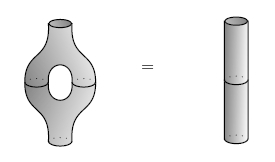and getting a new symmetric monoidal category $Cospan$ in which this new relation holds, together with a symmetric monoidal functor $2Cob \to Cospan$.

Do you know whether anyone has thought about the multi-object non-special case, i.e. a universal property for “labeled 2-cobordisms” among monoidal categories in which each object has a (non-special) commutative Frobenius algebra structure?

It doesn’t leap to mind. I can imagine certain TQFT experts or ‘topological string theory’ experts who could be interested in this, but I haven’t actually seen them write about this.

Posted by: John Baez on August 1, 2017 8:31 AM | Permalink | Reply to this

### Re: A Compositional Framework for Reaction Networks

Hmm, maybe decategorification is the wrong word. Dimension reduction? I feel like it’s saying we have these cobordisms relating circles and we just squash all the circles down to points and the cobordisms down to lines, in the process losing information about the genus.

Posted by: Mike Shulman on August 1, 2017 10:28 AM | Permalink | Reply to this

### Re: A Compositional Framework for Reaction Networks

Do you know whether anyone has thought about the multi-object non-special case, i.e. a universal property for “labeled 2-cobordisms”; among monoidal categories in which each object has a (non-special) commutative Frobenius algebra structure?

Walters has thought about the non-special case a bit. I’m not sure if the result you’re looking for is anywhere, but I might start with his paper Tangled Circuits and go from there. It also seems he might have called these categories reticular categories, but a search for that turned up nothing.

Posted by: Brendan Fong on August 9, 2017 6:31 PM | Permalink | Reply to this

### Re: A Compositional Framework for Reaction Networks

Wow! So we’re working on different aspects of the same thing.

I’ve been planning to write a paper called something like ‘Functorial semantics of open Petri nets’. Computer scientists are very fond of Petri nets without ‘rate constants’ labelling reactions — those rate constants are what makes the subject count as chemistry instead of computer science, though by now there’s a fascinating overlap, the field of ‘chemical computing’. Computer scientists think of a Petri net as a simple sort of program, and they’ve studied various kinds of semantics for Petri nets. In the process, Meseguer and Montanari made the crucial realization that (modulo some subtleties regarding strictness) a Petri net can be seen as a presentation of a symmetric monoidal category that is free on some objects (the species) and some morphisms (the reactions).

They seem, however, to have been slow in thinking about open Petri nets, and the symmetric monoidal category $Petri$ with open Petri nets as morphisms. So, I’ve been planning to describe various semantics for Petri nets as various symmetric monoidal functors $F : Petri \to X$, where $X$ is something like the category of sets and functions, or the category of sets and relations. The more ‘chemical’ kinds of semantics, like those discussed in my paper with Blake, require the reactions in the Petri net to be equipped with rate constants.

Reactions in chemistry are indeed a lot like deductions, with various species or propositions coming in (as ‘reactants’ or ‘hypotheses’) and going out (as ‘products’ or ‘conclusions’). What logicians call a ‘sequent’, mathematical chemists call a ‘complex’.

I hope we can talk more about this stuff. There aren’t many people who can think about the ‘logic of life’ with a sufficient background in both categorical logic and chemistry to exploit all the analogies.

I can start by looking over your papers. If you’re curious about what computer scientists have done with Petri nets (since their work is a bit closer to logic than the chemical stuff I’ve been focusing on), you might try Chapter 25 of my book with Jacob Biamonte. It’s called ‘Computation and Petri nets’, and you don’t need to have read the previous 24 chapters.

Posted by: John Baez on August 1, 2017 8:03 AM | Permalink | Reply to this

### Re: A Compositional Framework for Reaction Networks

I’d love to talk more about this stuff, although I haven’t thought about chemistry since probably my freshman year of college. I’ll try to find some time to have a look at that book chapter.

Posted by: Mike Shulman on August 1, 2017 10:34 AM | Permalink | Reply to this

### Re: A Compositional Framework for Reaction Networks

I know the relevant chemistry, so you don’t need to know about that. The book chapter is more up your alley: it’s about stuff computer scientists do with Petri nets.

For example, the reachability problem. Given a symmetric monoidal category $C$ free on some objects and some morphisms, and given two objects $x, y \in C$, is there a morphism from $x$ to $y$? This is decidable, but ‘just barely’: the run-time of the best known algorithm is not primitive recursive. The story of this is quite amusing and not completely finished.

Posted by: John Baez on August 1, 2017 10:52 AM | Permalink | Reply to this

### Re: A Compositional Framework for Reaction Networks

I just wanted to point out ongoing work on event structures by Glynn Winskel and others (including myself, to some extent), going back to the 1980s but still very active. Event structures are, roughly speaking, unrolled Petri nets. The point is that various different loop structures in Petri nets are often equivalent, semantically speaking, and a canonical representation is found by unrolling the loops to get an infinite structure, which is roughly what event structures are.

I mention all this because spans also play a crucial role in the work on compositional semantics using event structures. (See e.g. Relations in concurrency, or the more recent work on concurrent games and strategies.)

There are also connections to cubical sets via higher-dimensional automata, but that’s another story.

Anyway there’s a lot of interesting stuff here in this post and the discussion, thanks.

Posted by: Sam Staton on August 2, 2017 7:18 AM | Permalink | Reply to this

### Re: A Compositional Framework for Reaction Networks

How would you use this to describe an oscillating chemical reaction like the Belousov-Zhabotinsky Reaction?

http://cenblog.org/the-safety-zone/2011/09/chemical-oscillations-the-belousov-zhabotinsky-reaction/

I also thought of using this to represent biodiversity within an ecosystem, where the species represent different species of plants, animals, and predators and prey. The reactions would include animals eating plants, predators eating prey, animals and plants dying for reasons other than being eaten, such as starvation, and animals and plants reproducing.

Posted by: Jeffery Winkler on August 2, 2017 10:48 PM | Permalink | Reply to this

### Re: A Compositional Framework for Reaction Networks

The theory of reaction networks has been deeply studied, and oscillations are one big topic of interest. A bit more on that in my comment below. All Blake and I are doing is introducing a compositional framework that allows us to study reaction networks ‘one piece at a time’. A question for this new compositional framework is: what kinds of pieces need to be stuck together to obtain oscillatory behavior? This is not an easy question to settle in general, but there should be some interesting things one can say.

Reaction networks are widely used to study population biology and epidemiology as you suggest; for a bit more on that try my book:

Especially see sections 2.6–2.8 on the SI, SIR and SIRS models of infectious disease and section 6 on the Lotka–Volterra model of predator-prey interactions.

I also recommend this:

• Darren James Wilkinson, Stochastic Modelling for Systems Biology, Taylor and Francis, New York, 2006.
Posted by: John Baez on August 3, 2017 1:47 AM | Permalink | Reply to this

### Re: A Compositional Framework for Reaction Networks

Here’s a bit more about how our paper connects to other ideas in chemistry, written in response to a chemist’s questions:

There’s a tradition of studying chemical reactions in which concentrations of chemicals of obey a set of differential equations called the ‘rate equation’, based on the law of mass action: the rate of a chemical reaction is proportional to the concentrations of the reacting substances.

In real life all chemical reactions are reversible and a closed system tends to settle down into an equilibrium obeying detailed balance: the rate of any reaction is equal to that of the reverse reaction. However, in some cases the rate of some reverse reactions are so slow that it’s enlightening to consider what happens when they’re zero. Then we can have interesting phenomena, like solutions of the rate equation where the concentrations of chemicals are periodic as a function of time, or even chaotic. A famous example is the Belousov-Zhabotinsky reaction.

But the Belousov-Zhabotinsky reaction actually involves about 18 reactions, so it’s interesting to look for simpler model systems that display similar behavior. A famous one is the Brusselator. The reactions here are not reversible (they’re shown in the Wikipedia article I linked to), and the analysis is simplest when 2 of the 5 chemicals involved are treated as having concentrations so much higher than the rest that we can approximate these concentrations as fixed… even though these chemicals are getting used up.

This sort of thing led mathematical chemists to study chemical reaction networks where not all reactions are reversible and some concentrations are held fixed, or chemostatted.

The big revolution in chemical reaction network theory came in the 1960s, with the work of Aris, Feinberg, Jackson, Horn and others. They proved many theorems about the behavior of the rate equation for different classes of chemical reaction networks: existence, uniqueness or nonuniqueness of equilibrium solutions, periodic solutions, etc.

Around the 1970s, Prigogine focused attention on the nonequilibrium thermodynamics of ‘open’ systems, where for example chemicals are constantly being added and/or removed from a system. Then we can have ‘nonequilibrium steady states’, where there’s no change in chemical concentrations, but nonzero net flows: that is, a lack of detailed balance. Sometimes there exists a unique nonequilibrium steady state that is stable, but sometimes it becomes unstable and one sees oscillatory behavior. Prigogine developed a formalism to study this.

My work with Blake Pollard formalizes the notion of ‘open chemical reaction network’. We describe the processes that let you build a big open chemical reaction network from smaller pieces. We describe the laws obeyed by these ‘building up’ processes. We describe the ‘open rate equation’, a generalization of the rate equation for open chemical reaction networks. We describe how the open rate equation of a big open chemical reaction network is built up from the open rate equations of the pieces. We also describe how nonequilibrium steady states of a big open chemical reaction network are built up from nonequilibrium steady states of the pieces. The right language for describing all this is category theory, since that provides techniques for studying how open systems can be connected end-to-end (‘composition’) or in parallel (‘tensoring’).

Posted by: John Baez on August 3, 2017 3:34 AM | Permalink | Reply to this

### Re: A Compositional Framework for Reaction Networks

I recently started reading Joachim Kock’s Graphs, hypergraphs, and properads, and it seems like a pretty different approach than the formalism of decorated cospans, but perhaps with some related ideas. Like you, Kock is interested in formalizing (directed) graphs/hypergraphs “with open-ended edges”, and in decorating those graphs with extra information. As I understand it (but you’d probably be able to understand the paper much more quickly yourself), the high-level idea builds on Kock’s earlier work on polynomial functors and trees as well as his work with Joyal on “graphical species”: define appropriate categories of open (hyper)graphs, then study different notions of “decorated” (hyper)graphs as presheaves over a subcategory of “elementary” graphs (= connected graphs without inner edges, which can be listed up to isomorphism as the the “unit graph” with one edge and no nodes, together with the $(m,n)$-corollas). In particular, directed graphs/hypergraphs are considered as diagrams of finite sets of the form

$A \overset{s}\leftarrow I \overset{p}\rightarrow N \overset{q}\leftarrow O \overset{t}\rightarrow A$

with the condition that $s$ and $t$ are injective in the case of a directed graph, or alternatively that $\langle s,p\rangle : I \to A \times N$ and $\langle q,t\rangle : O \to N \times A$ are injective in the case of a directed hypergraph. (Here $N$ is the set of nodes, $A$ the set of (hyper)edges, $I$ and $O$ the set of in-flags and out-flags, and $\langle s,p\rangle$ and $\langle q,t\rangle$ the functions sending an oriented flag to a pair of an (hyper)edge and a vertex which are incident.)

Posted by: Noam Zeilberger on August 3, 2017 4:19 PM | Permalink | Reply to this

Post a New Comment